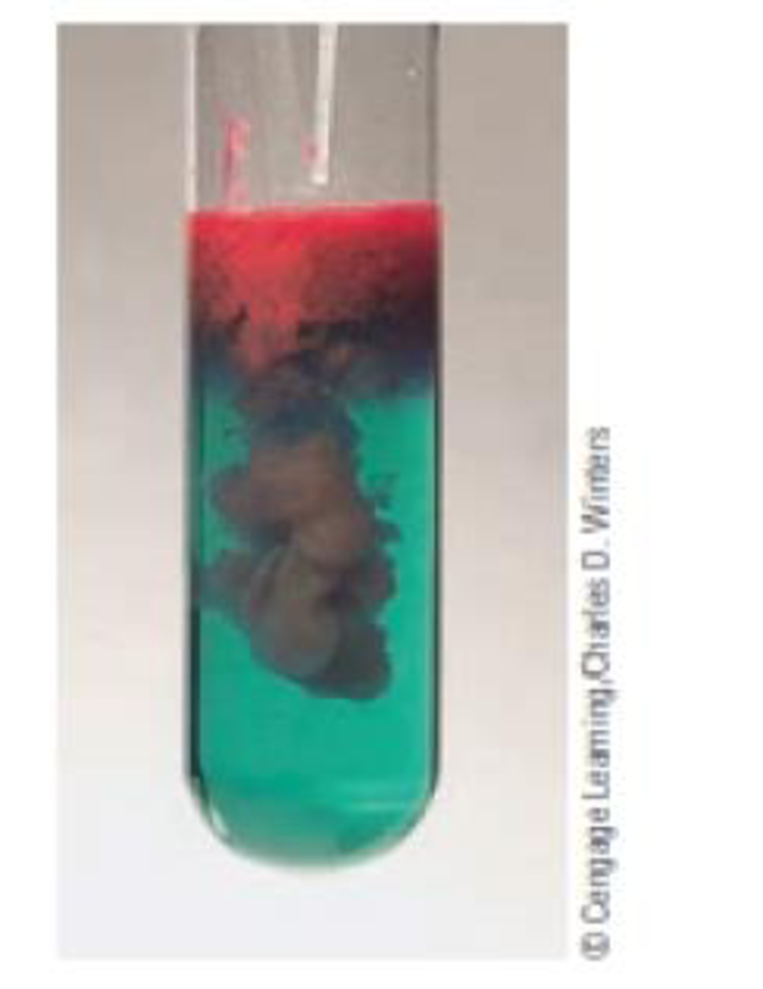Chapter 3, Problem 99SCQ

Chapter
Section
Textbook Problem

A common method for analyzing for the nickel content of a sample is to use a precipitation reaction. Adding the organic compound dimethylgly-oxime to a solution containing Ni2+ ions precipitates a red solid.Derive the empirical formula for the red solid based on the following composition: Ni, 20.315%; C, 33.258%; H, 4.884%; 0, 22.151%; and N, 19.392%.

Interpretation Introduction

Interpretation:

The empirical formula for the red solid based on the given composition should be derived.

Concept introduction:

Get the mass of each element by assuming a certain overall mass for the sample.  Convert the mass of each element to moles of each element using the atomic masses.  Find the ratio or the moles of each element by dividing the number of moles of each by the smallest number of moles.

Explanation

The molar mass of carbon is 12.00, the molar mass of nickel is 58.7, the molar mass of hydrogen is 1.00, the molar mass of oxygen is 16.0 and the molar mass of nitrogen is 14.0.

Therefore the empirical formula of the red solid is calculated below,

33.285 g ×1Molarmassofcarbon33.285 g ×112.00=2.7715

20.315 g ×1Molarmassofnickel20.315 g ×158.7=0.346

4.884 g ×1Molarmassofhydrogen4.884 g ×11=4.884

22.121 g ×1Molarmassofoxygen4

Still sussing out bartleby?

Check out a sample textbook solution.

See a sample solution

The Solution to Your Study Problems

Bartleby provides explanations to thousands of textbook problems written by our experts, many with advanced degrees!

Get Started